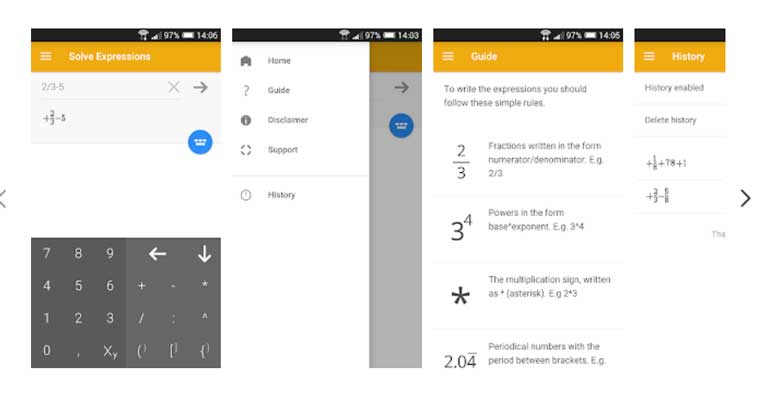# Top free apps to help you solve math exercises for Android

Do you need to quickly solve an equation or a math exercise?

You can install an app on your phone to help you find the solution quickly and see the step-by-step solving procedure.

Here are the best apps to help you solve math exercises, equations, and math problems for your Android phone or tablet.

## PhotoMath, the best math solving exercise app

One of the best apps to solve math exercises is Photomath, which allows you to solve mathematical exercises simply by taking a picture of them with your phone’s camera.

It’s very easy to use:

Install the app, open it, and tap the camera icon in the top menu.

Photograph the exercise to be solved and within seconds the application shows you the solution and the step-by-step solving procedure.

Alternatively, tap the calculator icon and type the equation you want to solve, and then tap the result to view the solving procedure.

## Solve Expressions, one of the best apps to solve math exercisesIt is extremely simple to use:

Open the app and in the main window add the exercise you want to solve.

The app, with the help of an automated algorithm, immediately suggests the result and how to solve it step by step.

You need to be connected to the Internet for everything to work well.

## All Math formula, mathematical formula app

All Math formula is not an exercise solving app, but it provides numerous mathematical formulas, over 1000.

Geometry formulas, trigonometry, algebra, vector calculations, probabilities, numerical methods and more are available.

The graphical interface is very intuitive and easy to use: just open the app and from the main window choose the package of formulas you want to look at.